# AP Physics B : Calculating Circuit Power

## Example Questions

### Example Question #1 : Calculating Circuit Power

Which of the following is not equivalent to a unit of power?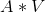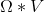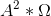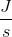Explanation:

The standard unit for power is Watts, but other equivalent units can be derived from the equations for power.

Remember that power is defined as work per unit time: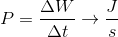The units from this equation will be Joules per second, eliminating that answer choice.

In terms of circuits, power is defined as the product of current and voltage, giving the units Ampere-Volts: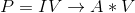If you combine Ohm’s law and the definition of power you can rewrite the equation for power as follows: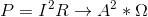These units will be Amperes squared times Ohms.

The only answer choice you are left with is Ohm-Volts. Power in terms of resistance and voltage is: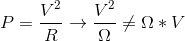### Example Question #2 : Calculating Circuit Power

Consider two resistors connected in series. Which of the following is true regarding its voltage, current, and power?

The voltage across both resistors is the same, but the larger resistor will have higher current and power dissipation

The current through both resistors is the same, but the smaller resistor will have higher voltage and power dissipation

The current through both resistors is the same, but the larger resistor will have higher voltage and power dissipation

The voltage across both resistors is the same, but the smaller resistor will have higher current and power dissipation

The current through both resistors is the same, but the larger resistor will have higher voltage and power dissipation

Explanation:

Since the resistors are connected in series, they will have the same current flowing through them. In series current is constant across resistors, and in parallel voltage is constant across resistors.

Remember the equations for voltage and power. The equation for voltage is Ohm's law: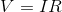Power can be written in terms of voltage and resistance, but in this problem only the current is held constant (because the resistors are in series) while the voltage of the resistors is different. The appropriate equation to use for comparison is: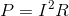From the equations you can deduce that increasing resistance will increase both voltage and power. The best answer is that current through both resistors is the same, but the larger resistor will have higher voltage and power dissipation.